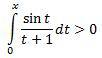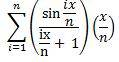# Integral inequality with sin proof

## Homework Statement

prove thatfor all x>0

## Homework Equations

-1 $$\leq$$ sin t $$\leq$$ 1

## The Attempt at a Solution

the area under the graph is increasing as x increases
also, i tried to write it the sigma way:then take the limit as n-->infinity
i got stuck trying to figure out how to work with sine in sigma notation, but i'm not even sure if my attempt would get anywhere

can anyone give me any pointers on how to do this?

## Answers and Replies

As x increases, when will the value of the integral be increasing, when will it be decreasing?